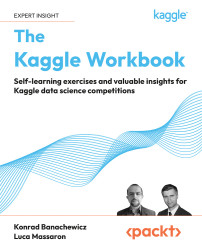•#### The Kaggle Workbook

5 (1)5 (1)

#### Overview of this book

More than 80,000 Kaggle novices currently participate in Kaggle competitions. To help them navigate the often-overwhelming world of Kaggle, two Grandmasters put their heads together to write The Kaggle Book, which made plenty of waves in the community. Now, they’ve come back with an even more practical approach based on hands-on exercises that can help you start thinking like an experienced data scientist. In this book, you’ll get up close and personal with four extensive case studies based on past Kaggle competitions. You’ll learn how bright minds predicted which drivers would likely avoid filing insurance claims in Brazil and see how expert Kagglers used gradient-boosting methods to model Walmart unit sales time-series data. Get into computer vision by discovering different solutions for identifying the type of disease present on cassava leaves. And see how the Kaggle community created predictive algorithms to solve the natural language processing problem of subjective question-answering. You can use this workbook as a supplement alongside The Kaggle Book or on its own alongside resources available on the Kaggle website and other online communities. Whatever path you choose, this workbook will help make you a formidable Kaggle competitor.
PrefaceFree Chapter
The Most Renowned Tabular Competition – Porto Seguro’s Safe Driver PredictionThe Makridakis Competitions – M5 on Kaggle for Accuracy and UncertaintyVision Competition – Cassava Leaf Disease CompetitionNLP Competition – Google Quest Q&A LabelingOther Books You May EnjoyIndex# Ensembling the results

Now, having two models, what’s left is to mix them together and see if we can improve the results. As suggested by Jahrer we go straight for a blend of them, but we do not limit ourselves to producing just an average of the two (since our approach in the end has slightly differed from Jahrer’s one) and we will also try to get optimal weights for the blend. We start importing the out-of-fold predictions and get our evaluation function ready:

``````import pandas as pd
import numpy as np
from numba import jit
@jit
def eval_gini(y_true, y_pred):
y_true = np.asarray(y_true)
y_true = y_true[np.argsort(y_pred)]
ntrue = 0
gini = 0
delta = 0
n = len(y_true)
for i in range(n-1, -1, -1):
y_i = y_true[i]
ntrue += y_i
gini += y_i * delta
delta += 1 - y_i
gini = 1 - 2 * gini / (ntrue * (n - ntrue))
return gini
``````

Once done, we convert the out-of-fold predictions of the LightGBM and the predictions of the neural network into ranks. We are doing so because the normalized Gini coefficient is based on rankings (as a ROC-AUC evaluation would be) and consequently blending rankings works better than blending the predicted probabilities::

``````lgb_oof_ranks = (lgb_oof.target.rank() / len(lgb_oof))
dnn_oof_ranks = (dnn_oof.target.rank() / len(dnn_oof))
``````

Now we just test if, by combining the two models using different weights, we can get a better evaluation of the out-of-fold data:

``````baseline = eval_gini(y_true=target.target, y_pred=lgb_oof_ranks)
print(f"starting from a oof lgb baseline {baseline:0.5f}\n")
best_alpha = 1.0
for alpha in [0.1, 0.2, 0.3, 0.4, 0.5, 0.6, 0.7, 0.8, 0.9]:
ensemble = alpha * lgb_oof_ranks + (1.0 - alpha) * dnn_oof_ranks
score = eval_gini(y_true=target.target, y_pred=ensemble)
print(f"lgd={alpha:0.1f} dnn={(1.0 - alpha):0.1f} -> {score:0.5f}")

if score > baseline:
baseline = score
best_alpha = alpha

print(f"\nBest alpha is {best_alpha:0.1f}")
``````

When ready, by running the snippet we can get interesting results:

``````starting from a oof lgb baseline 0.28850
lgd=0.1 dnn=0.9 -> 0.27352
lgd=0.2 dnn=0.8 -> 0.27744
lgd=0.3 dnn=0.7 -> 0.28084
lgd=0.4 dnn=0.6 -> 0.28368
lgd=0.5 dnn=0.5 -> 0.28595
lgd=0.6 dnn=0.4 -> 0.28763
lgd=0.7 dnn=0.3 -> 0.28873
lgd=0.8 dnn=0.2 -> 0.28923
lgd=0.9 dnn=0.1 -> 0.28916
Best alpha is 0.8
``````

It seems that blending a strong weight (0.8) on the LightGBM model and a weaker one (0.2) on the neural network will bring an even better-performing model. We immediately try this hypothesis by setting a blend of the same weights for the models and the ideal weights that we have found:

``````lgb_submission = pd.read_csv("../input/workbook-lgb/lgb_submission.csv")
"../input/porto-seguro-safe-driver-prediction/sample_submission.csv")
``````

First, we try the equal weights solution, which was the strategy used by Michael Jahrer:

``````lgb_ranks = (lgb_submission.target.rank() / len(lgb_submission))
dnn_ranks = (dnn_submission.target.rank() / len(dnn_submission))
submission.target = lgb_ranks * 0.5 + dnn_ranks * 0.5
submission.to_csv("equal_blend_rank.csv", index=False)
``````

It leads to a public score of 0.28393 and a private score of 0.29093, which is around 50th position on the final leaderboard, a bit far from our expectations. Now let’s try using the weights that the out-of-fold predictions helped us to find:

``````lgb_ranks = (lgb_submission.target.rank() / len(lgb_submission))
dnn_ranks = (dnn_submission.target.rank() / len(dnn_submission))
submission.target = lgb_ranks * best_alpha +  dnn_ranks * (1.0 - best_alpha)
submission.to_csv("blend_rank.csv", index=False)
``````

Here the results lead to a public score of 0.28502 and a private score of 0.29192, which turns out to be around the seventh position on the final leaderboard. A much better result indeed because the LightGBM is a good model, but it is probably missing some nuances in the data that can be provided by adding some information from the neural network trained on the denoised data.

Exercise 6

As pointed out by CPMP in their solution (https://www.kaggle.com/competitions/porto-seguro-safe-driver-prediction/discussion/44614), depending on how to build your cross-validation, you can experience a “huge variation of Gini scores among folds.” For this reason, CPMP suggests decreasing the variance of the estimates by using many different seeds for multiple cross-validations and averaging the results.

As an exercise, try to modify the code we used to create more stable predictions, especially for the denoising autoencoder.

Exercise Notes (write down any notes or workings that will help you):